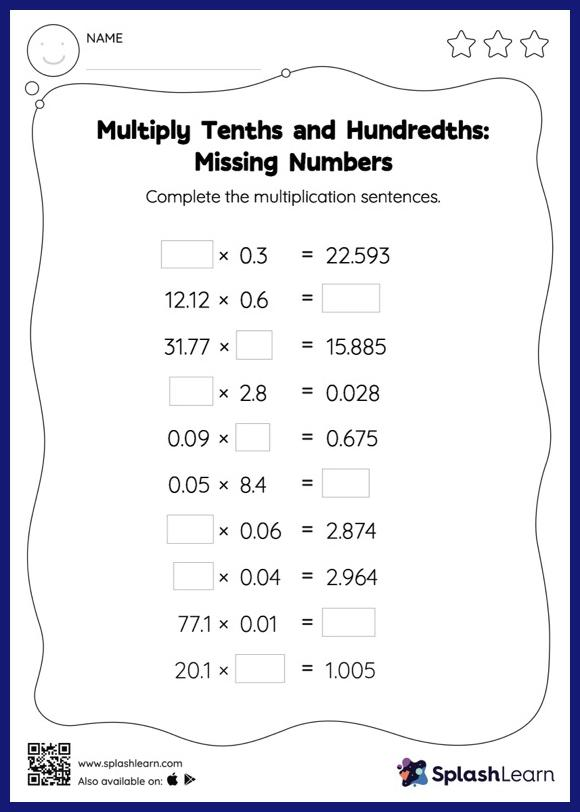# Multiply Tenths and Hundredths: 1-Digit Multiplication: Missing Numbers Worksheet

Home > Multiply Tenths and Hundredths: 1-Digit Multiplication: Missing NumbersThis multiply tenths and hundredths: 1-digit multiplication worksheet consists of a set of questions on multiplication, which help students develop fluency. When students multiply two decimals, they do so without considering the decimal point at first. They find the product and add the decimal point at the appropriate place in it. This approach helps them in finding the missing number in decimal multiplication as with multiply tenths and hundredths: 1-digit multiplication worksheet.